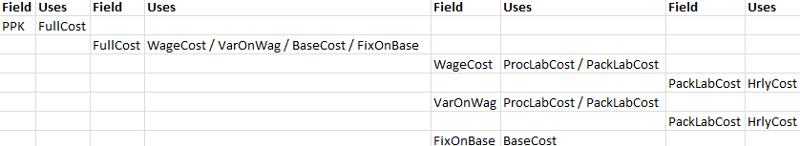SQL Query Help - find Field Name within Text - Iterative loopMark Damen used Ask the Experts™
on
Hi

I need some help to write an SQL query please.  I have a problem in my application where using calculated fields works fine when working with database fields or constants, and is also fine when a calculated field is used within another calculated field.  It break when there are nested calculated fields beyond 1 level deep.  I wish to construct a SQL query to identify to me any records which this affects.

Here is a sample of some rows where the problem exists:

TableID	FieldName	Seq	DataType	IsCalculated	Formula	FieldFormat	FieldLabel	Alias
Calculated	HrlyCost	8	decimal	1	50
Calculated	VarOnWag	14	decimal	1	(ProcLabCost + PackLabCost) * 999	->>,>>9.99	Variable on Wages	Calculated_VarOnWag
Calculated	FixOnBas	18	decimal	1	BaseCost * 999	->>,>>9.99	Fixed on Base	Calculated_FixOnBas
Calculated	FullCost	19	decimal	1	WageCost + VarOnWag + BaseCost + FixOnBas	->>,>>9.99	Fully Costed	Calculated_FullCost
Calculated	PackLabCost	12	decimal	1	(HrlyCost * Part_UD.Number07) / NULLIF((Part_UD.Number06 * 999), 0)	->>,>>9.99	Packing Labour Cost	Calculated_PackLabCost
Calculated	BaseCost	17	decimal	1	CostPart.StdMaterialCost * 150 / NULLIF(100, 0)	->>,>>9.99	Base Cost incl Waste	Calculated_BaseCost
Calculated	ProcLabCost	11	decimal	1	Part1_UD.Number05 * Part.NetWeight	->>,>>9.99	Process Labour Cost	Calculated_ProcLabCost
Calculated	WageCost	13	decimal	1	ProcLabCost + PackLabCost	->>,>>9.99	Wages Cost	Calculated_WageCost
Calculated	PPK	22	decimal	1	FullCost / NULLIF(Part.NetWeight, 0)	->>,>>9.99	Cost PPK	Calculated_PPK

I'll also try to demonstrate the nested fields here:The field PPK as you can see above has 4 levels.

Is there anyway to query for and show the number of levels based on the data provided above?

Many Thanks
Mark.
Comment
Watch Question

Do more withEXPERT OFFICE® is a registered trademark of EXPERTS EXCHANGE®
Top Expert 2014

Commented:
This lines up the column data better:
TableID 	FieldName	Seq	DataType	IsCalculated	Formula                                                         	FieldFormat 	FieldLabel          	Alias
Calculated	HrlyCost	8	decimal 	1           	50
Calculated	VarOnWag	14	decimal 	1           	(ProcLabCost + PackLabCost) * 999                               	->>,>>9.99  	Variable on Wages   	Calculated_VarOnWag
Calculated	FixOnBas	18	decimal 	1           	BaseCost * 999                                                  	->>,>>9.99  	Fixed on Base       	Calculated_FixOnBas
Calculated	FullCost	19	decimal 	1           	WageCost + VarOnWag + BaseCost + FixOnBas                       	->>,>>9.99  	Fully Costed        	Calculated_FullCost
Calculated	PackLabCost	12	decimal 	1           	(HrlyCost * Part_UD.Number07) / NULLIF((Part_UD.Number06 * 999), 0)	->>,>>9.99  	Packing Labour Cost 	Calculated_PackLabCost
Calculated	BaseCost	17	decimal 	1           	CostPart.StdMaterialCost * 150 / NULLIF(100, 0)                 	->>,>>9.99  	Base Cost incl Waste	Calculated_BaseCost
Calculated	ProcLabCost	11	decimal 	1           	Part1_UD.Number05 * Part.NetWeight                              	->>,>>9.99  	Process Labour Cost 	Calculated_ProcLabCost
Calculated	WageCost	13	decimal 	1           	ProcLabCost + PackLabCost                                       	->>,>>9.99  	Wages Cost          	Calculated_WageCost
Calculated	PPK     	22	decimal 	1           	FullCost / NULLIF(Part.NetWeight, 0)                            	->>,>>9.99  	Cost PPK            	Calculated_PPK
Most Valuable Expert 2014
Awarded 2013

Commented:
The string "PPK" is referenced 3 times, all in the same row of data, so I fail to see what you state I should.
Commented:
Hi,

SQL is smart, but its not that smart, so it is unreasonable to assume that the nesting is infinite like you are doing.

What you could do, is use a subquery where PPK and the next level are calculated, making them constants or fields in the next higher level query.

HTH
David

PS Often calculating things like the above are best left to the presentation layer ie SSRS reports or Crystal reports and so forth and so on, which may be more forgiving, whereas SQL is the data retrieval layer ...
Top Expert 2014

Commented:
What third party database tools are installed?
IT Engineer
Distinguished Expert 2017

Commented:
markusdamenous, do you still need help with this question?
ERP System Manager
Commented:
I didn't get a solution to this question here, but will award points to the answers that suggested alternatives.

The application provided has acknowledged the error that caused me to need to find the nested queries in the first instance, and I manually went though about 400 calculated fields and changed the formula.
ERP System Manager

Commented:
See my last comment for explanation of points allocation.

Do more withSubmit tech questions to Ask the Experts™ at any time to receive solutions, advice, and new ideas from leading industry professionals.